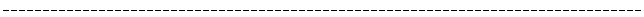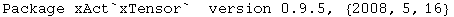This loads the package from the default directory, for example \$Home/.Mathematica/AddOns/Applications/xAct/ for a single-user installation under Linux. xTensor` and xPerm` are automatically loaded.

In:=Out=In:=

<<xAct`xCoba`Comparing, we see that the packages take about 10Mb  in Mathematica 5.2:

In:=Out=In:=Out=There are six contexts: xAct`xCoba`, xAct`xTensor`, xAct`xPerm` and xAct`ExpressionManipulation` contain the respective reserved words. System` contains Mathematica's reserved words. The current context Global` will contain your definitions and right now it is empty.

In:=Out=In:=Out=In:=We turn off the annoying spell messages:

In:=Created by Mathematica  (May 16, 2008)